• 这个程序是图像小波变换MatLab源代码，是用小波变换进行图像多分辨率分析的实用的例子。 这个程序是图像小波变换MatLab源代码，是用小波变换进行图像多分辨率分析的实用的例子。
• 小波变换matlab！！！！！！！！！！！！！！！！！！！！！！！！！！！！！！！！！！！！！！！！！！！！！！！！！！！！！！！！！！！！！！！！！！！！！！！！！！！！！！！！！！！！！！！！！！！！...
• DWT小波变换MATLAB 里面有图片 亲测可用
• 基于小波变换的去噪处理程序，matlab开发环境，可以立即运行。
• csi信道状态信息 利用matlab提取CSI后 对数据预处理 小波变换matlab代码 直接可用
• 调Q小波变换 MATLAB程序，亲测可用，好评一下哦，谢谢。
• 小波变换的原理所谓的小波的小是针对傅里叶波而言，傅里叶波指的是在时域空间无穷震荡的正弦(或余弦波)。相对而言，小波指的是一种能量在时域非常集中的波，它的能量有限，都集中在某一点附近，而且积分的值为零，这...

小波变换的原理
所谓的小波的小是针对傅里叶波而言，傅里叶波指的是在时域空间无穷震荡的正弦(或余弦波)。
相对而言，小波指的是一种能量在时域非常集中的波，它的能量有限，都集中在某一点附近，而且积分的值为零，这说明它与傅里叶波一样是正交波。
举一些小波的例子：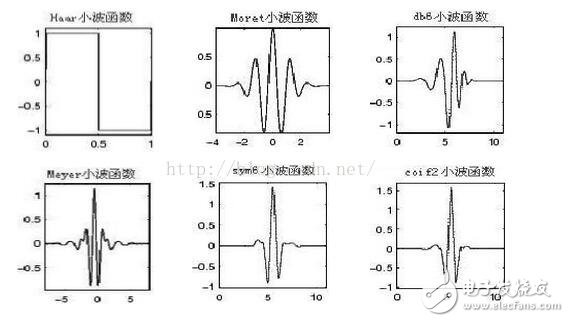可以看到，能量集中在x轴0值附近，以y轴的0值为基线，上下两个区域的波形面积相等。
众所周知，图像的傅里叶变换是将图像信号分解为各种不同频率的正弦波。同样，小波变换是将图像信号分解为由原始小波位移和缩放之后的一组小波。
小波在图像处理里被称为图像显微镜，原因在于它的多分辨率分解能力可以将图片信息一层一层分解剥离开来。剥离的手段就是通过低通和高通滤波器，
这里我们以一个图像的横向一维为例，讲讲小波的分解与还原，采用的是Haar小波做分解：
图像原始像素矩阵：［6 4 8 7 5 9 3 2］
分解低通滤波器：［ 1 1］/sqrt(2)
分解高通滤波器：［-1 1］/sqrt(2)
1、用低通滤波器与原始像素矩阵做卷积得：［8 10 12 15 12 14 12 5］/sqrt(2)
下采样得：［10 15 14 5］/sqrt(2) -----》L
2、用高通滤波器与原始像素矩阵做卷积得：［-4 2 -4 1 2 -4 6 1］/sqrt(2)
下采样得：［2 1 -4 1］/sqrt(2) -----》H
上例为一维情况，二维情况在做完横向滤波之后再进行纵向滤波即可。
二、逆变换过程：
重构低通滤波器：［1 1］/sqrt(2)
重构高通滤波器：［1 -1］/sqrt(2)
1、对L数组插值得：［0 10 0 15 0 14 0 5］/sqrt(2)
再用低通滤波器做卷积得：［10 10 15 15 14 14 5 5］/2
2、对H数组插值得：［0 2 0 1 0 -4 0 1］/sqrt(2)
再用高通滤波器做卷积得：［2 -2 1 -1 -4 4 1 -1］/2
两个数组求和得：［6 4 8 7 5 9 3 2］ ，矩阵被还原了。
三、基于小波变换的图像压缩
我们知道，图像的低频部分保存的是图像的轮廓信息，而高频保存的是图像的边缘和细节信息，大量的研究表明，幅值低的高频信息对于图像共享较小，
丢弃对图像质量的影响不大，所以小波变换的特性给了图像压缩一个很好的工具，将原图进行小波分解以后，为高频信息设置一个阈值a，假如该点的值小于a则置零这样就抛弃掉了图像中影响不大的低幅值高频信息，还原出来的图像没有明显的质量下降，但是占用空间却变小了。
给一个别人论文里的示例和统计：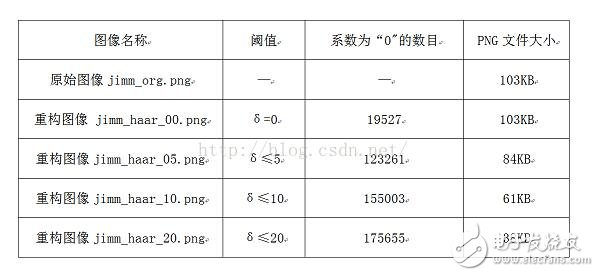效果：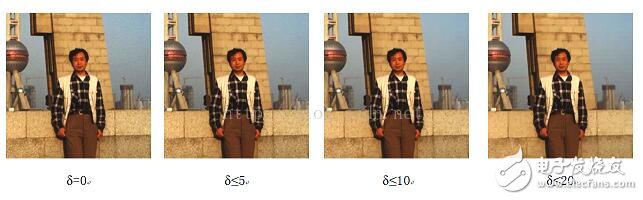图像小波变换的matlab实现详解
1、 一维小波变换的 Matlab 实现
(1) dwt 函数
功能：一维离散小波变换
格式：［cA，cD］=dwt(X，‘wname’)
［cA，cD］=dwt(X，Lo_D，Hi_D)
说明：［cA，cD］=dwt(X，‘wname’) 使用指定的小波基函数 ‘wname’ 对信号 X 进行分解，cA、cD 分别为近似分量和细节分量；［cA，cD］=dwt(X，Lo_D，Hi_D) 使用指定的滤波器组 Lo_D、Hi_D 对信号进行分解。
(2) idwt 函数
功能：一维离散小波反变换
格式：X=idwt(cA，cD，‘wname’)
X=idwt(cA，cD，Lo_R，Hi_R)
X=idwt(cA，cD，‘wname’，L)
X=idwt(cA，cD，Lo_R，Hi_R，L)
说明：X=idwt(cA，cD，‘wname’) 由近似分量 cA 和细节分量 cD 经小波反变换重构原始信号 X 。
‘wname’ 为所选的小波函数
X=idwt(cA，cD，Lo_R，Hi_R) 用指定的重构滤波器 Lo_R 和 Hi_R 经小波反变换重构原始信号 X 。
X=idwt(cA，cD，‘wname’，L) 和 X=idwt(cA，cD，Lo_R，Hi_R，L) 指定返回信号 X 中心附近的 L 个点。
2、二维小波变换的 Matlab 实现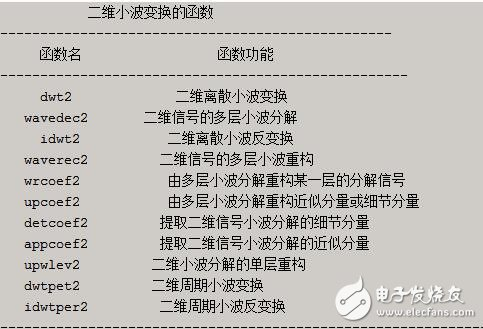(1) wcodemat 函数
功能：对数据矩阵进行伪彩色编码
格式：Y=wcodemat(X，NB，OPT，ABSOL)
Y=wcodemat(X，NB，OPT)
Y=wcodemat(X，NB)
Y=wcodemat(X)
说明：Y=wcodemat(X，NB，OPT，ABSOL) 返回数据矩阵 X 的编码矩阵 Y ；NB 伪编码的最大值，即编码范围为 0～NB，缺省值 NB＝16；
OPT 指定了编码的方式(缺省值为 ‘mat’)，即：
OPT＝‘row’ ，按行编码
OPT＝‘col’ ，按列编码
OPT＝‘mat’ ，按整个矩阵编码
ABSOL 是函数的控制参数(缺省值为 ‘1’)，即：
ABSOL＝0 时，返回编码矩阵
ABSOL＝1 时，返回数据矩阵的绝对值 ABS(X)
(2) dwt2 函数
功能：二维离散小波变换
格式：［cA，cH，cV，cD］=dwt2(X，‘wname’)
［cA，cH，cV，cD］=dwt2(X，Lo_D，Hi_D)
说明：［cA，cH，cV，cD］=dwt2(X，‘wname’)使用指定的小波基函数 ‘wname’ 对二维信号 X 进行二维离散小波变幻；cA，cH，cV，cD 分别为近似分量、水平细节分量、垂直细节分量和对角细节分量；［cA，cH，cV，cD］=dwt2(X，Lo_D，Hi_D) 使用指定的分解低通和高通滤波器 Lo_D 和 Hi_D 分解信号 X 。
(3) wavedec2 函数
功能：二维信号的多层小波分解
格式：［C，S］=wavedec2(X，N，‘wname’)
［C，S］=wavedec2(X，N，Lo_D，Hi_D)
说明：［C，S］=wavedec2(X，N，‘wname’) 使用小波基函数 ‘wname’ 对二维信号 X 进行 N 层分解；［C，S］=wavedec2(X，N，Lo_D，Hi_D) 使用指定的分解低通和高通滤波器 Lo_D 和 Hi_D 分解信号 X 。
(4) idwt2 函数
功能：二维离散小波反变换
格式：X=idwt2(cA，cH，cV，cD，‘wname’)
X=idwt2(cA，cH，cV，cD，Lo_R，Hi_R)
X=idwt2(cA，cH，cV，cD，‘wname’，S)
X=idwt2(cA，cH，cV，cD，Lo_R，Hi_R，S)
说明：X=idwt2(cA，cH，cV，cD，‘wname’) 由信号小波分解的近似信号 cA 和细节信号 cH、cH、cV、cD 经小波反变换重构原信号 X ；X=idwt2(cA，cH，cV，cD，Lo_R，Hi_R) 使用指定的重构低通和高通滤波器 Lo_R 和 Hi_R 重构原信号 X ；X=idwt2(cA，cH，cV，cD，‘wname’，S) 和 X=idwt2(cA，cH，cV，cD，Lo_R，Hi_R，S) 返回中心附近的 S 个数据点。
(5) waverec2 函数
说明：二维信号的多层小波重构
格式：X=waverec2(C，S，‘wname’)
X=waverec2(C，S，Lo_R，Hi_R)
说明：X=waverec2(C，S，‘wname’) 由多层二维小波分解的结果 C、S 重构原始信号 X ，‘wname’ 为使用的小波基函数；X=waverec2(C，S，Lo_R，Hi_R) 使用重构低通和高通滤波器 Lo_R 和 Hi_R 重构原信号。
图像处理工具箱1. 图像和图像数据
缺省情况下，MATLAB将图像中的数据存储为双精度类型(double)，64位浮点
数，所需存储量很大；MATLAB还支持另一种类型无符号整型(uint8)，即图像矩
阵中每个数据占用1个字节。
在使用MATLAB工具箱时，一定要注意函数所要求的参数类型。另外，uint8
与double两种类型数据的值域不同，编程需注意值域转换。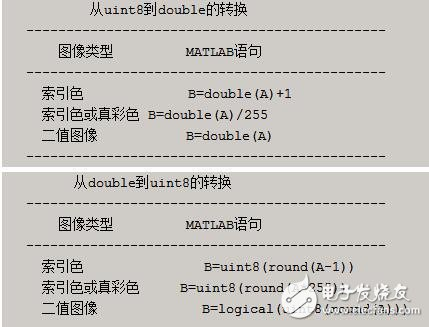3、图像处理工具箱所支持的图像类型
3.1、真彩色图像
R、G、B三个分量表示一个像素的颜色。如果要读取图像中(100，50)处的像素值，
可查看三元数据(100，50，1:3)。
真彩色图像可用双精度存储，亮度值范围是［0，1］；比较符合习惯的存储方法是用无
符号整型存储，亮度值范围［0，255］
3.2、索引色图像
包含两个结构，一个是调色板，另一个是图像数据矩阵。调色板是一个有3列和若干行
的色彩映象矩阵，矩阵每行代表一种颜色，3列分别代表红、绿、蓝色强度的双精度数。
注意：MATLAB中调色板色彩强度［0，1］，0代表最暗，1代表最亮。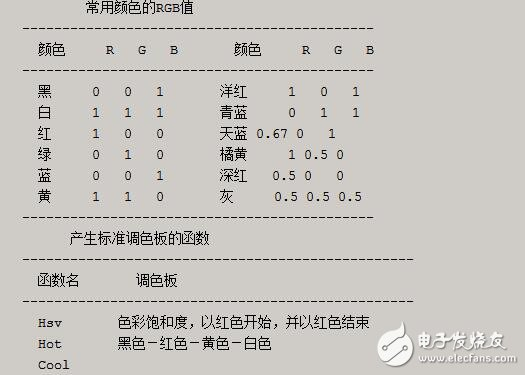展开全文• 小波变换的图像处理%MATLAB2维小波变换经典程序 % FWT_DB.M; % 此示意程序用DWT实现二维小波变换 % 编程时间2004-4-10，编程人沙威 %%%%%%%%%%%%%%%%%%%%%%%%%%%% %%%%%%%%%%%%%%%%%%%%%%%%%%% clear; ...
•  % 列变换 row(1:SUB_T,i)=dyaddown( ifft( fft(l_zeros).*fft(f(:,i)') ) ).'; % 圆周卷积FFT row(SUB_T+1:T,i)=dyaddown( ifft( fft(h_zeros).*fft(f(:,i)') ) ).'; % 圆周卷积FFT end; for j=1:T; % 行...

clear;clc;
T=256;       %  图像维数
SUB_T=T/2;   %  子图维数
%%%%%%%%%%%%%%%%%%%%%%%%%%%%%%%%%
%%%%%%%%%%%%%%%%%%%%%%%%%%%%%%%%%
%  1.调原始图像矩阵
f=X;         %  原始图像
%%%%%%%%%%%%%%%%%%%%%%%%%%%%%%%%
%%%%%%%%%%%%%%%%%%%%%%%%%%%%%%%%
%  2.进行二维小波分解
l=wfilters('db4','l');    %  db10(消失矩为10)低通分解滤波器冲击响应(长度为20)
L=T-length(l);
l_zeros=[l,zeros(1,L)];    %  矩阵行数与输入图像一致，为2的整数幂
h=wfilters('db4','h');    %  db10(消失矩为10)高通分解滤波器冲击响应(长度为20)
h_zeros=[h,zeros(1,L)];    %  矩阵行数与输入图像一致，为2的整数幂
for i=1:T;   %  列变换
row(1:SUB_T,i)=dyaddown( ifft( fft(l_zeros).*fft(f(:,i)') ) ).';    %  圆周卷积FFT
row(SUB_T+1:T,i)=dyaddown( ifft( fft(h_zeros).*fft(f(:,i)') ) ).';  %  圆周卷积FFT
end;
for j=1:T;   %  行变换
line(j,1:SUB_T)=dyaddown( ifft( fft(l_zeros).*fft(row(j,:)) ) );    %  圆周卷积FFT
line(j,SUB_T+1:T)=dyaddown( ifft( fft(h_zeros).*fft(row(j,:)) ) );  %  圆周卷积FFT
end;
decompose_pic=line;  %  分解矩阵
%  图像分为四块
lt_pic=decompose_pic(1:SUB_T,1:SUB_T);      %  在矩阵左上方为低频分量--fi(x)*fi(y)
rt_pic=decompose_pic(1:SUB_T,SUB_T+1:T);    %  矩阵右上为--fi(x)*psi(y)
lb_pic=decompose_pic(SUB_T+1:T,1:SUB_T);    %  矩阵左下为--psi(x)*fi(y)
rb_pic=decompose_pic(SUB_T+1:T,SUB_T+1:T);  %  右下方为高频分量--psi(x)*psi(y)
%%%%%%%%%%%%%%%%%%%%%%%%%%%%%%%%%%%%%%%%%%%
%%%%%%%%%%%%%%%%%%%%%%%%%%%%%%%%%%%%%%%%%%%
%  3.分解结果显示
figure(1);
colormap(map);
subplot(2,1,1);
image(f);  %  原始图像
title('原始图像');
subplot(2,1,2);
image(abs(decompose_pic));  %  分解后图像
title('分解后图像');
figure(2);
colormap(map);
subplot(2,2,1);
image(abs(lt_pic));  %  左上方为低频分量--fi(x)*fi(y)
title('逼近图像');
subplot(2,2,2);
image(abs(rt_pic));  %  矩阵右上为--fi(x)*psi(y)
title('水平细节');
subplot(2,2,3);
image(abs(lb_pic));  %  矩阵左下为--psi(x)*fi(y)
title('垂直细节');
subplot(2,2,4);
image(abs(rb_pic));  %  右下方为高频分量--psi(x)*psi(y)
title('对角细节');
%%%%%%%%%%%%%%%%%%%%%%%%%%%%%%%%%%%%%%%%%%%
%%%%%%%%%%%%%%%%%%%%%%%%%%%%%%%%%%%%%%%%%%%%
%  5.重构源图像及结果显示
% construct_pic=decompose_matrix'*decompose_pic*decompose_matrix;
%%%%%%%%%%%%%%%%%%%%%%%%%%%%%%%%%%%%%%%%%%%%%%%%%%%%%%%%%%%%%%
l_re=l_zeros(end:-1:1);   %  重构低通滤波
l_r=circshift(l_re',1)';  %  位置调整
h_re=h_zeros(end:-1:1);   %  重构高通滤波
h_r=circshift(h_re',1)';  %  位置调整
%%%%%%%%%%%%%%%%%%%%%%%%%%%%%%%%%%%%%%%%%%%%%%%%%%%%%%%%%%%%%%%
top_pic=[lt_pic,rt_pic];  %  图像上半部分
t=0;
for i=1:T;  %  行插值低频
if (mod(i,2)==0)
topll(i,:)=top_pic(t,:); %  偶数行保持
else
t=t+1;
topll(i,:)=zeros(1,T);   %  奇数行为零
end
end;
for i=1:T;  %  列变换
topcl_re(:,i)=ifft( fft(l_r).*fft(topll(:,i)') )';  %  圆周卷积FFT
end;
%%%%%%%%%%%%%%%%%%%%%%%%%%%%%%%%%%%%%%%%%%%%%%%%%%%%%%%%%%%%%%
bottom_pic=[lb_pic,rb_pic];  %  图像下半部分
t=0;
for i=1:T;  %  行插值高频
if (mod(i,2)==0)
bottomlh(i,:)=bottom_pic(t,:);  %  偶数行保持
else
bottomlh(i,:)=zeros(1,T);       %  奇数行为零
t=t+1;
end
end;
for i=1:T; %  列变换
bottomch_re(:,i)=ifft( fft(h_r).*fft(bottomlh(:,i)') )';  %  圆周卷积FFT
end;
construct1=bottomch_re+topcl_re;  %  列变换重构完毕
%%%%%%%%%%%%%%%%%%%%%%%%%%%%%%%%%%%%%%%%%%%%%%%%%%%%%%%%%%%%
left_pic=construct1(:,1:SUB_T);   %  图像左半部分
t=0;
for i=1:T;  %  列插值低频
if (mod(i,2)==0)
leftll(:,i)=left_pic(:,t); %  偶数列保持
else
t=t+1;
leftll(:,i)=zeros(T,1);    %  奇数列为零
end
end;
for i=1:T;  %  行变换
leftcl_re(i,:)=ifft( fft(l_r).*fft(leftll(i,:)) );  %  圆周卷积FFT
end;
%%%%%%%%%%%%%%%%%%%%%%%%%%%%%%%%%%%%%%%%%%%%%%%%%%%%%%%%%%%%
right_pic=construct1(:,SUB_T+1:T);  %  图像右半部分
t=0;
for i=1:T;  %  列插值高频
if (mod(i,2)==0)
rightlh(:,i)=right_pic(:,t);  %  偶数列保持
else
rightlh(:,i)=zeros(T,1);      %  奇数列为零
t=t+1;
end
end;
for i=1:T; %  行变换
rightch_re(i,:)=ifft( fft(h_r).*fft(rightlh(i,:)) );  %  圆周卷积FFT
end;
%%%%%%%%%%%%%%%%%%%%%%%%%%%%%%%%%%%%%%%%%%%%%%%%%%%%%%%%%%
construct_pic=rightch_re+leftcl_re;  %  重建全部图像
%%%%%%%%%%%%%%%%%%%%%%%%%%%%%%%%%%%%%%%%%%%%%%%%%%%%
%  结果显示
figure(3);
colormap(map);
subplot(2,1,1);
image(f);  %  源图像显示
title('原始图像');
subplot(2,1,2);
image(abs(construct_pic));   %  重构源图像显示
title('重构后图像');
error=abs(construct_pic-f);  %  重构图形与原始图像误值
figure(4);
mesh(error);  %  误差三维图像
title('误差三维图像');

展开全文• 本文件为matlab代码，内容为用于图像处理的小波变换
• 可用于分析小波变换算法的matlab实现，方法简单，易于应用
• Matlab实现小波变换文章来源：不详 作者：佚名--------------------------------------------------------------------------------该文章讲述了Matlab实现小波变换应用MATLAB 小波变换 2010-01-11 20:513. 图像小波...

Matlab实现小波变换
文章来源：不详 作者：佚名
--------------------------------------------------------------------------------
该文章讲述了Matlab实现小波变换应用
MATLAB 小波变换 2010-01-11 20:51
3. 图像小波变换的 Matlab 实现函数 fft、fft2 和 fftn 分析
3.1 一维小波变换的 Matlab 实现
(1) dwt 函数   Matlab
功能：一维离散小波变换
格式：[cA,cD]=dwt(X,'wname')
[cA,cD]=dwt(X,Lo_D,Hi_D)别可以实现一维、二维和 N 维 DFT
说明：[cA,cD]=dwt(X,'wname') 使用指定的小波基函数 'wname' 对信号X 进行分解，cA、cD 分别为近似分量和细节分量；[cA,cD]=dwt(X,Lo_D,Hi_D) 使用指定的滤波器组 Lo_D、Hi_D 对信号进行分解。
(2) idwt 函数
功能：一维离散小波反变换
格式：X=idwt(cA,cD,'wname')
X=idwt(cA,cD,Lo_R,Hi_R)
X=idwt(cA,cD,'wname',L)函数 fft、fft2 和 fftn 分
X=idwt(cA,cD,Lo_R,Hi_R,L)
说明：X=idwt(cA,cD,'wname') 由近似分量 cA 和细节分量 cD 经小波反变换重构原始信号 X 。
'wname' 为所选的小波函数
X=idwt(cA,cD,Lo_R,Hi_R) 用指定的重构滤波器 Lo_R 和 Hi_R 经小波反变换重构原始信号 X 。
X=idwt(cA,cD,'wname',L) 和 X=idwt(cA,cD,Lo_R,Hi_R,L) 指定返回信号 X 中心附近的 L 个点。
1. 离散傅立叶变换的 Matlab实现
3.2 二维小波变换的 Matlab 实现
二维小波变换的函数别可以实现一维、二维和 N 维 DFT
-------------------------------------------------
函数名                函数功能
---------------------------------------------------
dwt2            二维离散小波变换
wavedec2       二维信号的多层小波分解
idwt2           二维离散小波反变换   Matlab
waverec2        二维信号的多层小波重构
wrcoef2          由多层小波分解重构某一层的分解信号
upcoef2          由多层小波分解重构近似分量或细节分量1. 离散傅立叶变换的 Matlab实现
detcoef2         提取二维信号小波分解的细节分量
appcoef2        提取二维信号小波分解的近似分量
upwlev2         二维小波分解的单层重构1. 离散傅立叶变换的 Matlab实现
dwtpet2         二维周期小波变换
idwtper2        二维周期小波反变换
-------------------------------------------------------------函数 fft、fft2 和 fftn 分
(1) wcodemat 函数
功能：对数据矩阵进行伪彩色编码函数 fft、fft2 和 fftn 分
格式：Y=wcodemat(X,NB,OPT,ABSOL)
Y=wcodemat(X,NB,OPT)
Y=wcodemat(X,NB)
Y=wcodemat(X)
说明：Y=wcodemat(X,NB,OPT,ABSOL) 返回数据矩阵 X 的编码矩阵 Y ；NB 伪编码的最大值，即编码范围为 0～NB，缺省值 NB＝16；
OPT 指定了编码的方式(缺省值为 'mat')，即：别可以实现一维、二维和 N 维 DFT
OPT＝'row' ，按行编码
OPT＝'col' ，按列编码
OPT＝'mat' ，按整个矩阵编码函数 fft、fft2 和 fftn 分
ABSOL 是函数的控制参数(缺省值为 '1')，即：
ABSOL＝0 时，返回编码矩阵
ABSOL＝1 时，返回数据矩阵的绝对值 ABS(X)1. 离散傅立叶变换的 Matlab实现
(2) dwt2 函数
功能：二维离散小波变换
格式：[cA,cH,cV,cD]=dwt2(X,'wname')
[cA,cH,cV,cD]=dwt2(X,Lo_D,Hi_D)
说明：[cA,cH,cV,cD]=dwt2(X,'wname')使用指定的小波基函数 'wname' 对二维信号 X 进行二维离散小波变幻；cA，cH,cV,cD 分别为近似分量、水平细节分量、垂直细节分量和对角细节分量；[cA,cH,cV,cD]=dwt2(X,Lo_D,Hi_D) 使用指定的分解低通和高通滤波器 Lo_D 和 Hi_D 分解信号 X 。1. 离散傅立叶变换的 Matlab实现
(3) wavedec2 函数
功能：二维信号的多层小波分解1. 离散傅立叶变换的 Matlab实现
格式：[C,S]=wavedec2(X,N,'wname')
[C,S]=wavedec2(X,N,Lo_D,Hi_D)
说明：[C,S]=wavedec2(X,N,'wname') 使用小波基函数 'wname' 对二维信号 X 进行 N 层分解；[C,S]=wavedec2(X,N,Lo_D,Hi_D) 使用指定的分解低通和高通滤波器 Lo_D 和 Hi_D 分解信号 X 。别可以实现一维、二维和 N 维 DFT
(4) idwt2 函数
功能：二维离散小波反变换函数 fft、fft2 和 fftn 分
格式：X=idwt2(cA,cH,cV,cD,'wname')
X=idwt2(cA,cH,cV,cD,Lo_R,Hi_R)
X=idwt2(cA,cH,cV,cD,'wname',S)别可以实现一维、二维和 N 维 DFT
X=idwt2(cA,cH,cV,cD,Lo_R,Hi_R,S)
说明：X=idwt2(cA,cH,cV,cD,'wname') 由信号小波分解的近似信号 cA 和细节信号 cH、cH、cV、cD 经小波反变换重构原信号 X ；X=idwt2(cA,cH,cV,cD,Lo_R,Hi_R) 使用指定的重构低通和高通滤波器 Lo_R 和 Hi_R 重构原信号 X ；X=idwt2(cA,cH,cV,cD,'wname',S) 和 X=idwt2(cA,cH,cV,cD,Lo_R,Hi_R,S) 返回中心附近的 S 个数据点。
(5) waverec2 函数
说明：二维信号的多层小波重构
格式：X=waverec2(C,S,'wname')
X=waverec2(C,S,Lo_R,Hi_R)
说明：X=waverec2(C,S,'wname') 由多层二维小波分解的结果 C、S 重构原始信号 X ，'wname' 为使用的小波基函数；X=waverec2(C,S,Lo_R,Hi_R) 使用重构低通和高通滤波器 Lo_R 和 Hi_R 重构原信号。
Allnodes   计算树结点 函数 fft、fft2 和 fftn 分
appcoef   提取一维小波变换低频系数
appcoef2   提取二维小波分解低频系数
bestlevt   计算完整最佳小波包树 别可以实现一维、二维和 N 维 DFT
besttree   计算最佳(优)树
*　 biorfilt   双正交样条小波滤波器组
biorwavf   双正交样条小波滤波器     Matlab
*　 centfrq   求小波中心频率
cgauwavf   Complex Gaussian小波
cmorwavf   coiflets小波滤波器 1. 离散傅立叶变换的 Matlab实现
cwt   一维连续小波变换
dbaux   Daubechies小波滤波器计算
dbwavf   Daubechies小波滤波器   dbwavf(W)    W='dbN'   N=1,2,3,...,50 别可以实现一维、二维和 N 维 DFT
ddencmp   获取默认值阈值(软或硬)熵标准
depo2ind   将深度-位置结点形式转化成索引结点形式
detcoef   提取一维小波变换高频系数     Matlab
detcoef2   提取二维小波分解高频系数
disp   显示文本或矩阵
drawtree   画小波包分解树(GUI) 别可以实现一维、二维和 N 维 DFT
dtree   构造DTREE类
dwt   单尺度一维离散小波变换
dwt2   单尺度二维离散小波变换 别可以实现一维、二维和 N 维 DFT
dwtmode   离散小波变换拓展模式
*　 dyadup   二元插值 1. 离散傅立叶变换的 Matlab实现
entrupd   更新小波包的熵值
fbspwavf   B样条小波
gauswavf   Gaussian小波     Matlab
get   获取对象属性值
idwt   单尺度一维离散小波逆变换
idwt2   单尺度二维离散小波逆变换
ind2depo   将索引结点形式转化成深度—位置结点形式
*　 intwave   积分小波数
isnode   判断结点是否存在      Matlab
istnode   判断结点是否是终结点并返回排列值
iswt   一维逆SWT(Stationary Wavelet Transform)变换
iswt2   二维逆SWT变换     Matlab
leaves   　 Determine terminal nodes
mexihat   墨西哥帽小波
meyer   Meyer小波 别可以实现一维、二维和 N 维 DFT
meyeraux   Meyer小波辅助函数
morlet   Morlet小波
nodease   计算上溯结点
nodedesc   计算下溯结点(子结点)
nodejoin   重组结点
nodepar   寻找父结点 别可以实现一维、二维和 N 维 DFT
nodesplt   分割(分解)结点
noleaves   　 Determine nonterminal nodes
ntnode   　 Number of terminal nodes函数 fft、fft2 和 fftn 分
ntree   　 Constructor for the class NTREE
*　 orthfilt   正交小波滤波器组
plot   绘制向量或矩阵的图形
*　 qmf   镜像二次滤波器
rbiowavf   　 Reverse biorthogonal spline wavelet filters
read   读取二进制数据 函数 fft、fft2 和 fftn 分
*　 scal2frq   　 Scale to frequency
set   　   Matlab
shanwavf   　 Shannon wavelets
swt   一维SWT(Stationary Wavelet Transform)变换
swt2   二维SWT变换
symaux   　 Symlet wavelet filter computation.
symwavf   Symlets小波滤波器
thselect   信号消噪的阈值选择
thodes   　 References
treedpth   求树的深度
treeord   求树结构的叉数   函数 fft、fft2 和 fftn 分
upcoef   一维小波分解系数的直接重构
upcoef2   二维小波分解系数的直接重构
upwlev   单尺度一维小波分解的重构 函数 fft、fft2 和 fftn 分
upwlev2   单尺度二维小波分解的重构
wavedec   单尺度一维小波分解
wavedec2   多尺度二维小波分解     Matlab
wavedemo   小波工具箱函数demo
*　 wavefun   小波函数和尺度函数
*　 wavefun2   二维小波函数和尺度函数 别可以实现一维、二维和 N 维 DFT
*　 wavemngr   小波管理函数
waverec   多尺度一维小波重构 1. 离散傅立叶变换的 Matlab实现
waverec2   多尺度二维小波重构
wbmpen   　 Penalized threshold for wavelet 1-D or 2-D de-noising
wcodemat   对矩阵进行量化编码 1. 离散傅立叶变换的 Matlab实现
wdcbm   　 Thresholds for wavelet 1-D using Birge-Massart strategy
wdcbm2   　Thresholds for wavelet 2-D using Birge-Massart strategy
wden   用小波进行一维信号的消噪或压缩
wdencmp   　De-noising or compression using wavelets
wentropy   计算小波包的熵
wextend   　Extend a vector or a matrix
*　 wfilters   小波滤波器
wkeep   提取向量或矩阵中的一部分
*　 wmaxlev   计算小波分解的最大尺度 1. 离散傅立叶变换的 Matlab实现
wnoise   产生含噪声的测试函数数据
wnoisest   估计一维小波的系数的标准偏差
wp2wtree   从小波包树中提取小波树    　1. 离散傅立叶变换的 Matlab实现
wpcoef   计算小波包系数
wpcutree   剪切小波包分解树
wpdec   一维小波包的分解 函数 fft、fft2 和 fftn 分
wpdec2   二维小波包的分解
wpdencmp   用小波包进行信号的消噪或压缩
wpfun   小波包函数 函数 fft、fft2 和 fftn 分
wpjoin   　重组小波包
wprcoef   小波包分解系数的重构
wprec   一维小波包分解的重构 1. 离散傅立叶变换的 Matlab实现
wprec2   二维小波包分解的重构
wpsplt   分割(分解)小波包
wpthcoef   进行小波包分解系数的阈值处理 函数 fft、fft2 和 fftn 分
wptree   　显示小波包树结构
wpviewcf   　 Plot the colored wavelet packet coefficients.
wrcoef   对一维小波系数进行单支重构 别可以实现一维、二维和 N 维 DFT
wrcoef2   对二维小波系数进行单支重构
wrev   向量逆序
write   向缓冲区内存写进数据
wtbo   　 Constructor for the class WTBO
wthcoef   一维信号的小波系数阈值处理
wthcoef2   二维信号的小波系数阈值处理 1. 离散傅立叶变换的 Matlab实现
wthresh   进行软阈值或硬阈值处理
wthrmngr   阈值设置管理
wtreemgr   管理树结构   Matlab

展开全文• 下面是离散二维(harr）小波变换MATLAB代码的实现： clear;clc; %%%%%%%%%%测试图像只能是方形图像，长宽像素一样。 f=imread('Lena.jpg');%%读取图像数据，图像在当前所在的路径下 d=size(f); if length(d)>2 f=...
由于图像是二维信号，二维小波变换应用到图像处理的基本思路是把小波变换有一维推广到二维。
下面是离散二维(harr）小波变换MATLAB代码的实现：
clear;clc;
%%%%%%%%%%测试图像只能是方形图像，长宽像素一样。
d=size(f);
if length(d)>2
f=rgb2gray((f));%%%%%%%%如果是彩色图像则转化为灰度图
end
T=d(1);
SUB_T=T/2;
%  2.进行二维小波分解
l=wfilters('db10','l');    %  db10（消失矩为10)低通分解滤波器冲击响应（长度为20）
L=T-length(l);
l_zeros=[l,zeros(1,L)];    %  矩阵行数与输入图像一致，为2的整数幂
h=wfilters('db10','h');    %  db10（消失矩为10)高通分解滤波器冲击响应（长度为20）
h_zeros=[h,zeros(1,L)];    %  矩阵行数与输入图像一致，为2的整数幂
for i=1:T;   %  列变换
row(1:SUB_T,i)=dyaddown( ifft( fft(l_zeros).*fft(f(:,i)') ) ).';    %  圆周卷积<->FFT
row(SUB_T+1:T,i)=dyaddown( ifft( fft(h_zeros).*fft(f(:,i)') ) ).';  %  圆周卷积<->FFT
end;
for j=1:T;   %  行变换
line(j,1:S
展开全文...

# 小波变换matlabmatlab 订阅